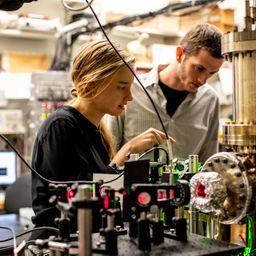Ask your homework questions to teachers and professors, meet other students, and be entered to win \$600 or an Xbox Series X 🎉Join our Discord!Numerade Educator

# Red light of wavelength 670 . nm produces photoelectrons from a certain photoemissive material. Green light of wavelength 520 . nm produces photoelectrons from the same material with 1.50 times the maximum kinetic energy. What is the material's work function?

## \text { Work Function of the material=0.7834419 }

Quantum Physics

Atomic Physics

### Discussion

You must be signed in to discuss.
##### Top Physics 103 Educators##### Christina K.

Rutgers, The State University of New Jersey### Video Transcript

um, you know it? Yes, yes, There is an easier way to do it. So I mean, so what? I was So let's write down the the original formula. So we know kinetic energy is equal to the energy of the protons. We sure knows HC over Lambda Week, but we can play that in later. So So it's just gonna be the energy of the proton minus, uh, the work function. And so if we know that, you know, like, can't you want you one and find one, and we know Well, actually, sacrifice caution to both so you can come up with that equation for the first situation and write down the equation for the second situation, and then we let's. Now, let's break down the givens. I'm gonna not. So let's just say we know you wanted me to just try to remember that you wanted me to are known. Well, go ahead and write that down you, but I'm not gonna write it down until the end. And then we also know that Katie, too, is equal to 1.5. Okay, He won. Let me just really the problem. Make sure that's correct. Yeah. So we're gonna call the Red Line one and then the green Line two. So we want to solve for if I So what we want to do is take you through these equations or actually, wind. Let's go ahead and take this. Plug it in here. So then we're left with 1.5 K one. I really wish it right faster with this pad, but it just gets murky, okay? Equals e to minus five. And, um then we have two equations to unknowns. These are the two equations and what we wanted Teoh. Right? We know. We know you want. We know it's you. We don't know Katie. One what we know, Fi. So what we can do is take this equation and just play it in here and solve for fi. So then we can say 1.5. So, Nike, you won, but rather he won minus bye. Because, Kate, you want to see Walter? You want minus five equals continuing with this bottom equation e to minus. Bye. So now we have 1.5 eyes on this. Identify that one. Fire on this side. Sorry. This is negative. 1.5. So we want to bring this. Um Why? Said the other side. So 1.5 minus one. Um, this 0.5. So you have a 0.5 fi on this side, and then we can bring the u two over. So then that's gonna be 1.5 you won minus E too, and can multiply way both sides by Chu to get if I get rid of this 1/2. So then that this one point if I was gonna become three, and then this is gonna become two. And then now we can go ahead and plug in the way. Blink So it's gonna be XY over Lambda one, My best to age. See over Lambda too. And then Lambda Wine, they say, is, um 6 70 then 5 20 when the Nano meter was, of course, time some of the minus nine. And then I'm gonna go ahead and plug this into a calculator. And so you see, So it's C kind one over 6 70 Nam, you knows 70. Thanks. 10 to the minus nine. Plus, we're not with minus 1/5. 20 10 to the minus nine Poon, we call it a negative number. We should not have bought a negative number. So let's figure out what happened. You some eligible wrong. 31 Um, that's to me too. A man? No, King Thio 1.5 key one, see? So Oh, I think I forgot my three into factor three two. Great. Now I have a positive number, and then I'm gonna go ahead and convert that to, uh you need a primal provided by 1.6 foot. You'd have the last 19 and then I got a 190.783 b.University of Washington

#### Topics

Quantum Physics

Atomic Physics

##### Top Physics 103 Educators##### Christina K.

Rutgers, The State University of New Jersey